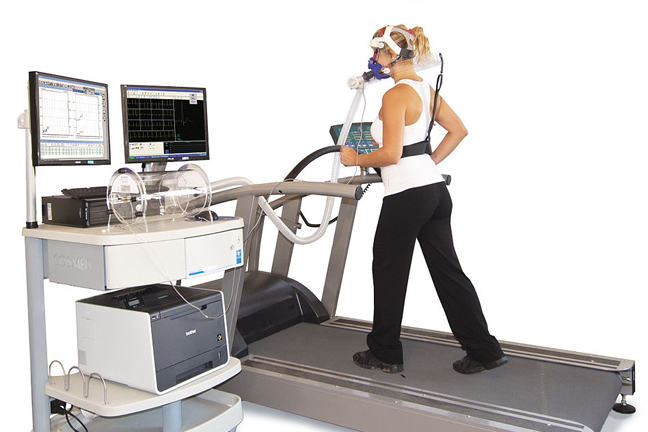## Want to keep learning?

This content is taken from the UNSW Sydney's online course, Maths for Humans: Linear, Quadratic & Inverse Relations. Join the course to learn more.
4.18

## UNSW SydneyErgospirometry laboratory

# Metabolic rate and Kleiber's law

Kleiber’s law was proposed by Max Kleiber, a Swiss chemist, in the 1930’s. The idea is to try to quantify the relation between how big an animal is, and what its total metabolic rate is. When we say big here, we mean mass. Metabolic rate is defined as energy per unit time, usually calories per second, and in this case is for the entire animal, not just a cell. So as the mass increases, so does the overall metabolic rate, but it is not a linear relation, but rather a power law.

In this step we look at metabolism and Kleiber’s law.

## The metabolism of an animal

Metabolism refers to the chemical processes that keep an animal alive. This involves breaking down incoming nutrients, and building up required compounds. It is a very complicated story. Since most chemical processes require oxygen, scientists can use oxygen consumption as a way of measuring metabolic rate. This is usually done when the animal is at rest.

We know that the mass of an animal scales cubically with its linear size, while the surface area scales quadratically. Since metabolism relates to the rate of input and output of materials (i.e. oxygen) of an organism, one might expect that metabolism scales proportionally to surface area, or to cross-sectional area.

This would suggest that the metabolic rate of an animal ought to be roughly proportional to the $$\normalsize{\frac{2}{3}}$$ power of its mass. Some early versions of Kleiber’s law stated the relation in this form.

## Kleiber’s law

But the modern form of Kleiber’s law states that an animal’s metabolic rate scales to the $$\normalsize{\frac{3}{4}}$$ power of its mass, that is

$\Large{\text{metabolic rate} \approx \operatorname{constant} \times \left(\operatorname{mass}\right)^\frac{3}{4}}.$

So a cat, having a mass $$\normalsize{100}$$ times that of a mouse, will metabolise roughly $$\normalsize{100^\frac{3}{4} = 31.6227766\cdots \approx 32}$$ times more energy per day than a mouse.

Q1. (E): Does this imply that a cat ought to consume 32 mice per day?

There is also a version of the law valid for plants, but there the exponent is close to $$\normalsize{1}$$; that is metabolic rate and mass are almost directly proportional.

Here is a diagram from Kleiber’s work. Note that to determine the power law nature of the relation the logarithm of metabolic rate is plotted against the logarithm of the mass. This is a standard technique when working with power laws. By using log scales on the axes, we can use our understanding of lines to understand more complicated power laws.

So the power law above can be rewritten as:

$\Large{\ln\left(\text{metabolic rate}\right) \approx \frac{3}{4}\ln\left(\operatorname{mass}\right)} + \ln\left(\operatorname{constant}\right).$Kleiber 1947 By Max Kleiber, Public domain, via Wikimedia Commons

Q2 (E): Can you see where the slope of $$\frac{3}{4}$$ appears in the above graph?

## Why does the exponent $$\normalsize{\frac{3}{4}}$$ appear?

Various explanations of the law have been given, but it seems that the biological community is not united in an explanation of the law, despite its wide range of applicability.

In fact there is a range of opinion on the validity of Kleiber’s law. While most scientists ascribe to this law, one might safely say that the greater the universality of application of the law, the wider is the range of skepticism. There is also some range of disagreement as to whether the best exponent is actually $$\normalsize{\frac{3}{4}}$$ or perhaps another value, and whether or not it depends on the species in question.

## Discussion

What other aspects of the story might we want to know about? Are there other points of view that we have not considered in this rather brief overview?